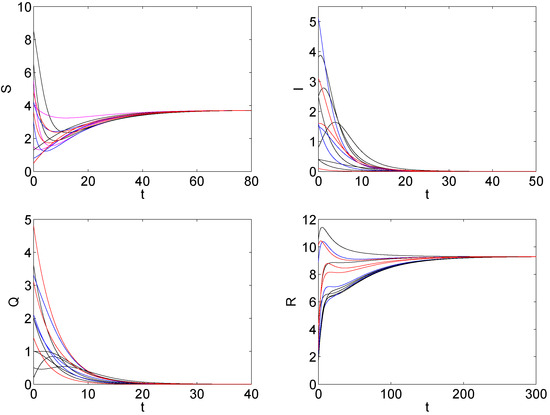Previous Article in Journal
Symmetric Radial Basis Function Method for Simulation of Elliptic Partial Differential Equations
Open AccessArticle

# Global Dynamics of an SIQR Model with Vaccination and Elimination Hybrid Strategies

byYanli Ma 1,Jia-Bao Liu 2,*andHaixia Li 1
1
Department of General Education, Anhui Xinhua University, Hefei 230088, China
2
School of Mathematics and Physics, Anhui Jianzhu University, Hefei 230601, China
*
Author to whom correspondence should be addressed.
Mathematics 2018, 6(12), 328; https://doi.org/10.3390/math6120328
Received: 18 October 2018 / Revised: 4 December 2018 / Accepted: 6 December 2018 / Published: 14 December 2018
(This article belongs to the Special Issue Mathematical Models in Epidemiology )
In this paper, an SIQR (Susceptible, Infected, Quarantined, Recovered) epidemic model with vaccination, elimination, and quarantine hybrid strategies is proposed, and the dynamics of this model are analyzed by both theoretical and numerical means. Firstly, the basic reproduction number $R 0$ , which determines whether the disease is extinct or not, is derived. Secondly, by LaSalles invariance principle, it is proved that the disease-free equilibrium is globally asymptotically stable when $R 0 < 1$ , and the disease dies out. By Routh-Hurwitz criterion theory, we also prove that the disease-free equilibrium is unstable and the unique endemic equilibrium is locally asymptotically stable when $R 0 > 1$ . Thirdly, by constructing a suitable Lyapunov function, we obtain that the unique endemic equilibrium is globally asymptotically stable and the disease persists at this endemic equilibrium if it initially exists when $R 0 > 1$ . Finally, some numerical simulations are presented to illustrate the analysis results. View Full-Text
Show FiguresFigure 1

MDPI and ACS Style

Ma, Y.; Liu, J.-B.; Li, H. Global Dynamics of an SIQR Model with Vaccination and Elimination Hybrid Strategies. Mathematics 2018, 6, 328.